# Count, CountIf, CountIfs in Excel

##### How to use Count, CountIf and CountIfs functions in MS Excel?

In the previous post, we learnt about Keyboard shortcuts in MS Excel. In this post, we shall learn how to count cells using Count, CountIf and CountIfs function.

### Count() function

Count() function is used to count the number cells between two ranges that contains numberical data; it doesn't count the cell containing string data.

Let's take below as an example. In 'I4', we have written '=COUNT(A4:G4)', it count 7 columns data.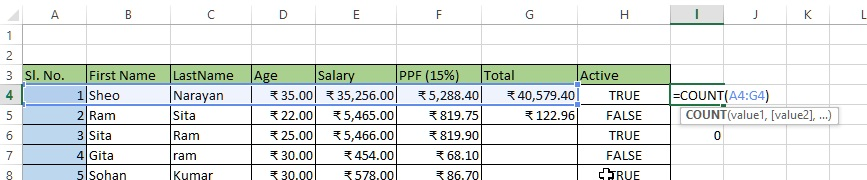The result is only 5 as shown below because only 5 columns (Sl. No., Age, Salary, PPF, Total) columns having numberical data.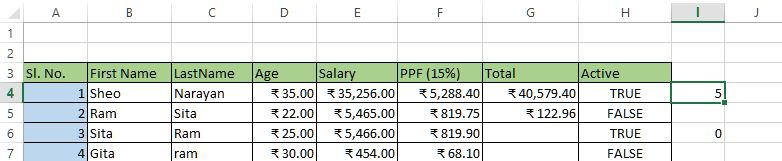Similarly, we are trying to count the rows that contains numberical data.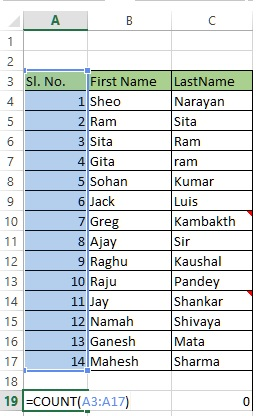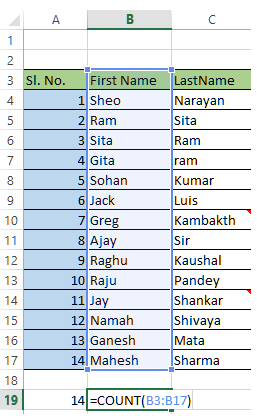As you can see that both A19 and B19 cell is using same formula to count row 3 to 17 but the result would be below.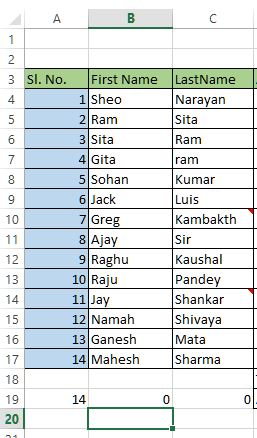As A column contains numberic data so it is giving number of rows, but B column has all string data so the result is 0.

### CountIf() function

CountIf function is used to count cells based on one criteria only (less than, greater than, equal to etc.). The criteria can be either for numerical value or string value.

Look at the below example. In D21 cell, the formula is written as '=COUNTIF(D4:D17, "<18")', it means that Count only those cells having value less than 18 and in this case only one cell D13 valid so the result we are getting is 1.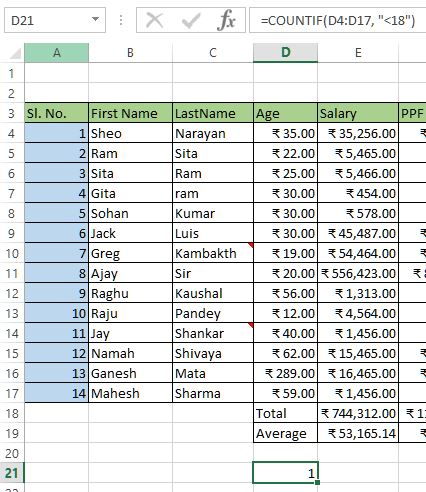We can even use wildcard characters in CountIf function.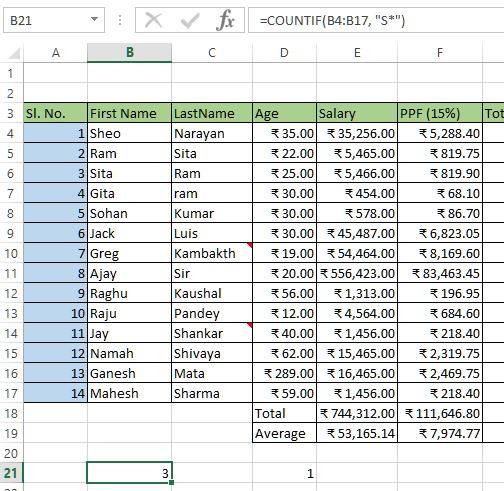This is counting cells whose string value starts with "S".

### CountIfs() function

CountIfs function is used to count cells based on multiple criteria (separated by comma). In below example, D21 cell contains '=COUNTIFS(B4:B17, "S*", D4:D17, "<26")' formula that says

• count those cells from B4:B17 (cell range) whose string starts with "S*" (this will give 3 records) and also
• D4:D17 cells whose value is '<26' (this will remove two records 'Sheo' & 'Sohan').
So if both criteria is met then only that particular record will be counted.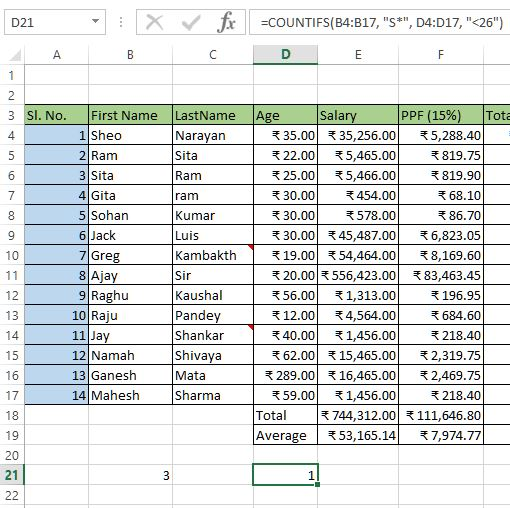So the result is 1.

### How to count cells with specific text in Excel?

To count cells with specific text in Excel, we can use CountIf() function. Select a blank cell and write formula like this '=COUNTIF(B4:B17, "Sheo")'. In this case I want to find 'Sheo' text.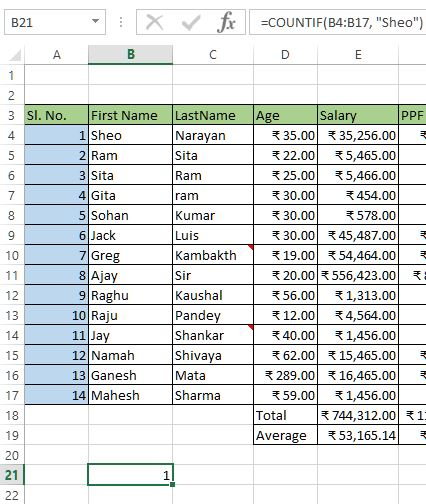and here is my result in B21.

As written earlier, we can use wild card characters also to count the occurences, like

1. Count cells starting with 'Sh' - '=COUNTIF(B4:B17, "Sh*")'
2. Count cells ending with 'eo' - '=COUNTIF(B4:B17, "*eo")'
3. Count cells contains 'h' - '=COUNTIF(B4:B17, "*h*")'
How to count non blank cells in Excel?

To count Non Blank cell in excel, use the same COUNTIF function but the 2nd parameter would be '<>'. So the formula to count non blank cells is '=COUNTIF(G4:G19, "<>")' where the range of cells it is counting is G4 to G19.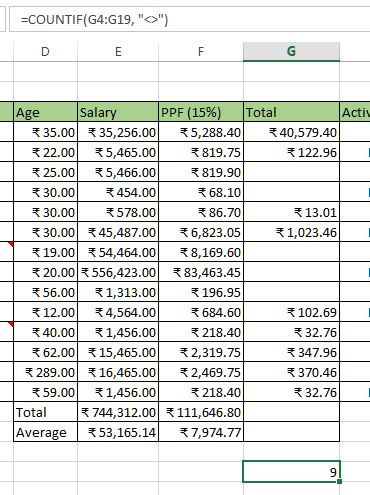See here non blank cell is 9.

### How to count blank cells in Excel?

To count blank cells in Excel, use the COUNTIF function with 2nd parameter as "". So here is the formula '=COUNTIF(G4:G19, "")'.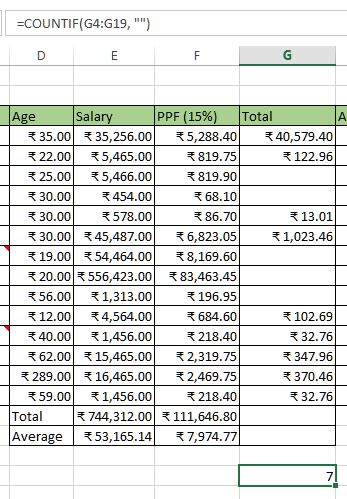The number of blank cells between G4 to G19 is 7.

Views: 6754 | Post Order: 16